﻿ Analytical Design Method of PI Regulators for First Order Plants ControlPublications are Open
Access in this journal
Article Versions
Export Article
• Normal Style
• MLA Style
• APA Style
• Chicago Style
Research Article
Open Access Peer-reviewed

### Analytical Design Method of PI Regulators for First Order Plants Control

Orlando Regalón Anias , Francisco Herrera Fernández, Milagros Diez Rodríguez, Douglas D. Crockett
Journal of Automation and Control. 2019, 7(1), 25-32. DOI: 10.12691/automation-7-1-4
Received August 15, 2019; Revised September 28, 2019; Accepted October 15, 2019

### Abstract

In this paper we propose a designing method for proportional-integral (PI) regulators to control first order plants. It’s based on the analytical expression of the control loop temporal response for a step input, obtained by using inverse Laplace transform. For the application of this method, the desired peak time and maximum overshoot are set as design specifications. The proposed design method is compared with a pole placement method, and adjustments are made to meet design specifications with a discrete implementation of the regulator. Finally, we shown the actual application and performance of this method in a direct current electric drive control.

### 1. Introduction

There are many different methods of designing proportional-integral-derivative (PID) controllers. On the one hand, we find the experimental method, among which is the widely used Ziegler-Nichols method 1 and its variants as proposed by Chien, Hrons and Reswick 2. They do not need to know the model of the plant, but only some characteristics of its response to predict signals. On the other hand, the analytical methods use a known mathematical model of the plant to reach the controller through mathematical operations. In the latter case, the direct design method, also known as the Ragazzini method 3, is found. There are also methods based on optimization that seek to minimize a function of tracking error and multiple variants 4.

A common goal pursued by all these methods to obtain a control system with stable operation in a closed loop. Several methods work on specifications in response to input signals, as in the optimal and symmetric module. Other methods indirectly seek the transient performance from various parameters. This is the case of methods based on frequency response, where characteristics such as bandwidth and phase margin are specified which are related to the speed of response and the oscillatority.

All of these methods, with their comparative advantages and disadvantages, have been well studied. They usually provide an acceptable fulfillment of the objectives in relation to the design specifications (i.e. the closed-loop dynamic behavior indexes). It is necessary, however, to continue investigating alternative methods to improve the accuracy of calculating the regulator parameters and to achieve a more precise connection between the indices of desired behavior and those that are actually obtained.

This is the goal of the research reported in this paper. Based on previously established values of behavioral indexes and based on previously established models, this paper calculates the PID regulator parameters in general, and the proportional-integral (PI) type in particular.

The paper is presented in the following form: after this introduction, a second section briefly discusses the design of regulators. A third section describes the plant that will be used as an example for the application of the proposed method, which is detailed with the exposition and demonstration of the analytical relations used in the fourth section. The fifth section compares the proposed method with the poles location method. Finally, section six present the conclusions and recommendations for continuing this type of research.

### 2. Regulator Design

In general, a feedback loop can be described by the diagram shown in Figure 1. The signal c(t) corresponds to the controlled output, and the inputs r(t), d(t) and n(t) represent the set point, load disturbance and noise in the measurement, respectively. Normally, the controller has the objective of generating the control signal m(t) to keep the error e(t) as close as possible to zero.

• Figure 1. General structure of a control system

The regulator can be of PID type or use any other structure, (e.g. delay-advance network). It can also use any transfer function, physically feasible, implemented in a discrete (or continuous), state-space control, adaptive control strategy, or employ artificial intelligence techniques.

In industrial practice, which includes both processes and electrical drives, more than 95% of the regulators used in the existing control loops are PIDs 2. Most programmable logic controllers (PLCs) have functions for implementing PID controllers. The current versions of Matlab also have Simulink blocks that behave as PID regulators with various structures, continuous or discrete, and with several variants of discretization in the latter case.

2.1. Design Specifications

When designing a control system, the purpose of the closed-loop system is to comply with the response to a given input with a set of specifications, both with regard to the transient stage and to the steady state stage corresponding to the response. The regulator needs to control the plant to ensure its operation.

Design specifications can be chosen with countless combinations, and many specifications are contradictory. For example, increasing the response speed increases the control effort; therefore, it is not possible to wish for the control system to behave quickly and with little control effort. This implies that a compromise must be reached between those specifications.

This paper addresses the application of an analytical design method of PI regulators which seeks to guarantee three specifications of dynamic performance that include:

• zero steady state error on step input,

• maximum overshoot specified, and

• specified peak time.

The first specification ensures zero tracking error of constant entries per tranches which is usually desired in most control systems. The second specification relates to the oscillatority of the transient stage, particularly with the relative maximum value that exceeds the response to a sudden change in input. The third specification determines the response speed.

The peak time has been chosen here instead of the establishment time because the former can be accurately determined analytically. In theory, the response of a dynamic system never reaches a final value, so different criteria such as 5% or 2% can be used to consider the system as steady state. In most cases, approximate expressions are used to find an exact analytical expression to calculate the establishment time, because calculating the exact is too complex 5.

### 3. The Control Object: CD Engine as First Order Plant

In order to facilitate the presentation of the proposed design method, a plant consisting of speed control under laboratory conditions will be defined in an armature controlled direct current motor with constant and independent excitation. This section provides a description of the installation, deriving the corresponding model, as well as the estimated parameters.

Figure 3 shows the assembly that makes up the control object. This includes the actuator which in this case is the direct current –alternating current converter (AC/DC), the actual plant formed by the motor–load assembly and the sensor. For convenience in performing the mathematical analysis later, the conditioned signal of the sensor is considered as output and not the speed in engineering units.

• Figure 2. Control object

The armature voltage is supplied by a single-phase, semi-controlled AC/DC converter which is governed by a control voltage m(t) from zero to ten volts DC and is supplied with 220 volts of AC. The motor shaft is mechanically coupled to a DC generator, which feeds a set of two variable resistive loads which can or cannot be connected. In this way, the load condition of the motor can be varied and thus cause soft and step-type disturbances.

In order to measure the output of the system c(t), a speed sensor coupled to a conditioning circuit is used which gives a voltage of zero to ten volts of DC between its output terminals. Note that the plant as a whole has a gain close to unity because of the way that input and output signals have been chosen.

3.1. The Mathematical Model

The mathematical model of this test plant is very simple and well known 5. Its transfer function, taking as input and output the signals indicated in Figure 2, is as follows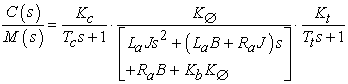(1)

where: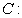voltage at the output of the speed sensor-signal conditioning, proportional to the speed of the motor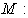control voltage of AC / DC converter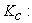AC / DC converter gain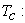time constant of the AC / DC converter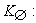motor torque constant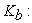counter-electromotive force constant of the motor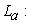armature inductance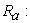motor armature resistance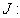inertia of the motor-load assembly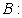friction of the motor-load assembly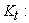speed sensor gain-signal conditioner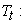time constant of the speed sensor

It can be seen that the dynamic model describing this plant is of the fourth order. In practice it happens that Tc, La and Tt are very small and can be considered equal to zero. This allows us to approximate the model of the set to a first order, which can be expressed as 6: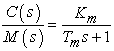(2)

where: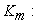system gain (in this case of the converter-motor-load-speed sensor)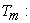system time constant (in this case also refers to said set).

Taking into account the above considerations, the parameters of this first order transfer function are calculated as follows: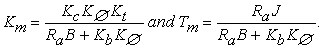(3)

As can be seen, the values of Km and Tm depend on seven parameters of the converted-motor-load-speed sensor set. Some parameters are easily measured, such as Kt and Ra. Other parameters are somewhat difficult to determine with the normally available instruments (as with J), or are also dependent on conditions of load and linearity (as with B).

3.2. Identification

Because it is difficult to calculate the values of Km and Tm, they must be estimated. This estimate can also validate the assumptions made to convert the fourth order model to a first order model. To complete an accurate identification, the static relationship between the input and output of the plant is first characterized and then the dynamic model is identified around an operation point.

By identifying the input-output characteristic, we can know the plant’s behavior in terms of linearity. This information is essential to design the test signals that identify the dynamic model.

To perform the static input-output characteristic identification, a double ramp was applied to the control signal m(t). This was designed with the characteristic that its slope was low enough to consider that, at any moment, the output of the system can be stable. It was also taken into account that the double ramp would cover the entire possible range of the input signal.

Two hardware variants were used to acquire the data pairs: a PLC from LG, MasterK-120S model with an analog input-output ADHA module, and a USB-NI-6212 data acquisition card from National Instrument. In the first case, the data was recorded in converter digital units (0 to 4000), while in the second case, the data was recorded in voltage (0 to 10 VDC).

Figure 3 shows the result obtained. Both axes have been scaled in relative values, which eliminates any dependence on units of the variant hardware used. The control and speed signals conditioners were adjusted to obtain maximum utilization of the measurement ranges. In engineering units, the actual engine speed varies between 0 and 1500 revolutions per minute (rpm). This causes a ratio of 0.375 rpm per converter units for the PLC case and 150 rpm per volt for the card.

• Figure 3. Static input-output characteristic of the control object

It is observed that there is no difference between when the command signal is increasing and it is decreasing, indicating that there is no hysteresis. Although noise is present in the measurement, the signal-to-noise ratio is very favorable.

On the other hand, we can see the severe nonlinearity presented by the study set, including a noticeable dead zone, followed by a sudden jump. This is due to two elements: the drive of a CD engine has a dead zone product of its starting torque which is usually non-zero; the trip circuit has also been designed to cause an increase in the dead zone and the appearance of a jump. This severe nonlinearity is the object of study in other investigations. In the upper part of the characteristic there is a tendency to saturation. There is a considerable space where the relationship is linear.

For the identification of the system there is a methodology widely used today known as pseudo random binary sequences (PRBS) which is based on the application of signal type 7. These signals have an autocorrelation function very similar to a Dirac delta, so they are very useful to estimate the impulsive response and to auto-correlate the response signal of the system under study.

The first step to perform the identification using this technique is to design and generate the PRBS signal, and it is important to take into account the bandwidth of the dynamic system under study. For this determination, an elementary nonparametric identification was applied with a step signal, and the response was observed. This process allowed us to identify the model from it’s estimated bandwidth which, in this case, was approximately 10 radians per second (1.6 Hz). The sampling frequency was chosen from 50 Hz, meeting the practical criterion of being greater than ten times the bandwidth. The sampling time was, therefore, 20 milliseconds.

For this case a PRBS of order 6 was designed with two consecutive complete sequences, which allows us to use a first session for identification and a second session for validation. The levels were chosen of 0.5 and 0.7 per unit; which fall into the linear part of the set’s input-output characteristic. The PRBS was defined with 10 samples per state which, at the defined sampling time, corresponds to 200 milliseconds of duration for each simple state. Thus, the fastest changes are in the order of a constant time, while the slowest changes allow the response to stabilize. The total experiment time was 25.4 seconds. The applied PRBS signal and the response of the plant under study are shown in Figure 4.

• Figure 4. Identification experiment applying signal type PRBS

From the data obtained, more than twenty possible models were estimated, combining different structures and orders. We compared the similarity coefficient and the correlation of the residuals calculated by Matlab for each case 8. Finally, taking into account the knowledge of the dynamic behavior of the plant, we chose the model that most faithfully reproduces the output from the input signal which was: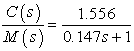(4)

This result corroborates that the approximations made are valid, since the first order model of (4) proved to be a better representation of the dynamic system behavior than the higher order models.

### 4. Analytical Design Method

As explained in the introduction, this paper concentrates on PID regulators. First, the design of the controller for a continuous control system will be analyzed. Although different structures exist, the ideal PID regulator is described from the following expression 9: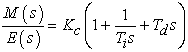(5)

where:constant of proportional action [normally dimensionless].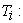integral action time [s]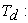derivative action time [s]

As a control object, a first order dynamic system with no transport delay and zero is selected which is defined by (2). For this type of plant with P or PD regulators it is not possible to achieve zero steady state error for step input. For this reason, we will not work with these variants of the PID controller. In this paper only the PI variant will be analyzed, leaving the PID variant for future research.

The general block diagram of Figure 1, with a PI regulator and the first order plant, is shown in Figure 5:

• Figure 5. First order plant controlled by a PI regulator

The closed-loop transfer function, which describes the effect of the input R(s) on the output C(s), is given by: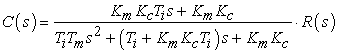(6)

For a step input R(s) = 1/s: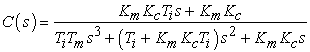(7)

If it defined: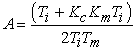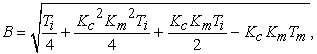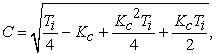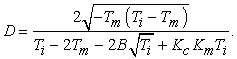(8)

Finding the inverse Laplace transform gives the analytical expression of the temporal response c(t):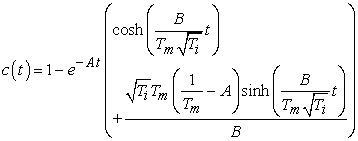(9)

c(t) it´s derivate for find de t value where the response is maximum: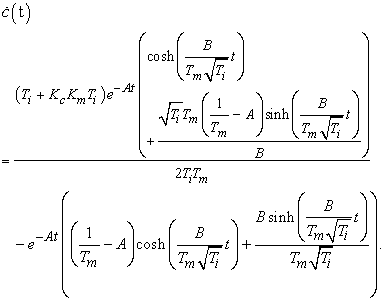(10)

Finding the value of t for which it is true that ċ(t) = 0 gives the expression for the peak time: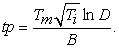(11)

Substituting this value of t into (9) gives the maximum value of the transient response. Subtracting 1, its relative value is obtained: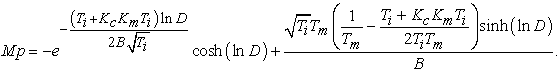(12)

Now a system of two non-linear equations with two unknowns is formed. To do this, equalize (11) at the desired peak time and (12) at the specified maximum overshoot. The unknowns to be found are the values of Kc and Ti satisfying these two equations.

For example, for the values of Km and Tm identified, shown in (4), for a desired peak time of 0.3 seconds and a desired maximum overshoot of 10%, we have the following system of two non-linear equations with two unknowns: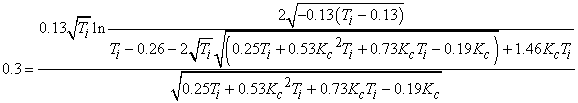(13)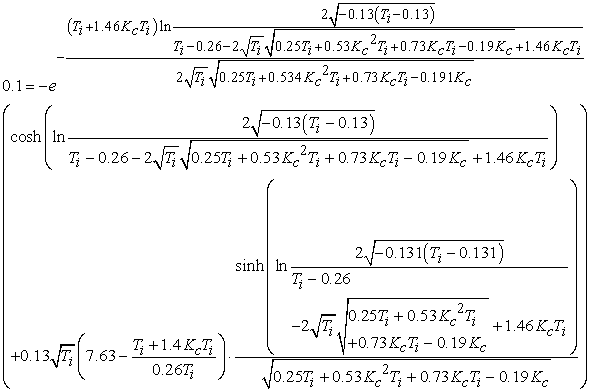(14)

Solving these equations determines the values of Kc and Ti required to meet the previously established design specifications.

### 5. Application of the Method

In this section the proposed method for designing the PI controller is applied in the installation control loop described earlier. For the purpose of comparison, a controller design is first presented using the known poles placement method. Then the method proposed in this work is used, and finally, a comparison of the results is presented.

5.1. Design by Poles Placement

From the diagram shown in Figure 5 the closed loop transfer function can alternatively be expressed as: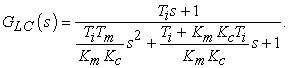(15)

Clearly, the closed-loop control system meets the specification to guarantee zero tracking error at the closed-loop step input. The question is: what values should Kc and Ti have so that the closed-loop performance is characterized by a given overshoot and peak time?

The values of overshoot and peak time are well defined for a second order system which has no zeros. This is not the case, since GLC(s) has a zero. In order to simplify the exposure of the subsequent application of the method, the effect of this zero will be considered negligible, and the following approximation will be made: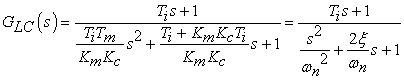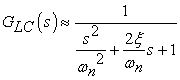(16)

where: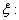damping ratio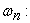undamped natural frequency

Equating the corresponding coefficients of the characteristic equation, the following system of equations is obtained: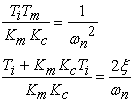(17)

The values of ξ and ωn are determined by the overshoot design specifications Mp and peak time tp. Here it is important to note that, for the response of a second-order system to have overshoot and peak time, it must be satisfied that ξ<1. This implies that the roots of the characteristic equation are complex conjugate. From the expressions for the calculation of Mp and tp, the following equations can be obtained 5: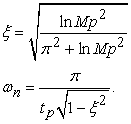(18)

Solving the system of equations given by 17 gives: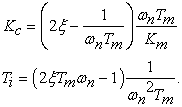(19)

It is important to note that any combination of peak time and maximum overshoot can be achieved in principle, but to have a positive Kc and Ti, it must satisfy: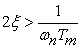(20)

Otherwise, negative values would be obtained, implying that the regulator will have a zero on the right side of plane s. The temporal response in this case will correspond to that of a non-minimal phase system with an erratic initial part.

Before a step input and in order to translate these values into a second-order system, the value of ωn can be substituted for the value derived from the settling time calculation with 2% criterion.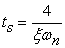(21)

Isolating ωn from here and substituting it in (20), we get positive values of Kc and Ti so it follows that: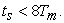(22)

This means that for a desired overshoot, it has to choose a peak time such that by defining this an ωn, it is true (22).

For example, this method of designing a PI regulator will be applied to the identified plant given by (4). The design specifications are to obtain a maximum overshoot of 10% (Mp = 0.1) and a peak time tp of 0.3 seconds, for a step input. Using the expressions given in (18), we calculate that a second-order system (without zero) must have a ξ≈0.59 and a ωn13 rad/s. Using the 2% criterion, the establishment time is 0.52 seconds, calculated according to (21). This satisfies the condition given in (22) so that positive values will be obtained for Kc and Ti, and the closed loop system will have a minimum phase behavior.

It also means that, the regulator for the control system of Figure 5 will be designed to obtain the following closed loop transfer function: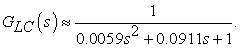(23)

Known Km and Tm, ξ and ωn, the expressions given in 19 are used and Kc=0.8056 and Ti=0.0506 seconds are obtained. Figure 6 shows the simulation results of the control system’s behavior which is achieved by applying this design method (discontinuous blue) and comparing it to the desired response of the defined design specifications (continuous red). The difference in the responses is observed because the zero that the PI contributed to the closed-loop transfer function was not taken into account during the design. This zero increases the response speed (making the peak time smaller) and increases the maximum overshoot value.

The response obtained in the sampling instants also graphs a discrete implementation of the controller (black asterisks). This further deflects the peak time and the maximum overshoot of the values specified for the design.

• Figure 6. Comparison between the desired response and that achieved with the design by the poles placement method

This poles placement method, as its name implies, guarantees the location of the roots of the characteristic equation, but it has no control over the possible zeros that will appear. Something similar happens with the existing variant of this method for the control in space of states 5.

5.2. The Proposed Analytical Design Method

To apply the proposed method, it is only necessary to solve (13) and (14), whereby Kc=0.64 and Ti=0.0601 seconds are obtained. Obviously, it is not easy to find the solution manually, but using Matlab makes this process easy.

Figure 7 shows the desired response (continuous red) and the achieved response with the proposed analytical design method (discontinuous blue). It is observed that they are very similar and particularly, have equal peak time and maximum overshoot values which were the specified performance parameters.

• Figure 7. Comparison between the desired response and that achieved with the design by the proposed analytical method

This last graph (Figure 7) also presents the response of a control system with discrete PI regulator, including the adjustments calculated by the proposed method. Although it improves with respect to that shown in Figure 6, the response still deviates from the desired response. The effect of discreetly implementing the regulator does not exactly meet the design specifications.

5.3. Adjustments of the Proposed Analytical Method to Achieve the Design Specifications with Discrete Implementation

With what has been seen so far, compliance with the design specifications that have been defined is guaranteed by using an analogical controller as shown in Figure 1. In current practice it is highly likely that the regulator will be performed digitally.

In order to implement the regulator in a computational element it is necessary to obtain a discrete equivalence of its continuous transfer function which is given by (5). There are several ways to obtain a discrete equivalence, among them are the one that equates the location of poles and zeros, which guarantees equal impulse response (transform table), or equal response to step (zero order hold), or equal Response to the ramp (first order hold), etc. Also the methods of the approximation of the integral are found 3.

There are three methods that approximate the integral of the discrete and continuous signals: forward, backward and trapezoidal. The latter is also known as Tustin, and is often used to approximate analog filters in their discrete equivalent because it can match the frequency response of both implementations for a given frequency. It cannot be used to approximate regulators. Using the forward equivalence, the left part of the complex plane s is transformed into the area to the left of the line z=1 in the z plane. This makes a stable continuous dynamic system that can be transformed into an unstable discrete equivalence. The backward equivalence does not have this drawback, hence it is the most commonly used method to obtain a discrete PID 10.

To apply the backward equivalence, the following substitution is performed in the continuous transfer function: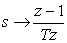(24)

Here s and z are the continuous and discrete complex frequencies respectively and T is the sampling interval expressed in seconds.

Making this substitution in (5) and operating algebraically we have: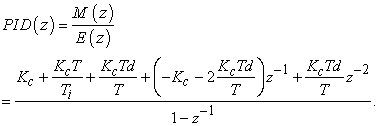(25)

For the case of a PI, where Td = 0, there remains: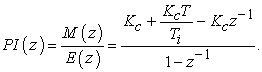(26)

To ensure that design specifications are met with the discrete implementation of the controller, an algorithm of successive approximations is proposed. To do this, the peak time is adjusted so that they are equal in the continuous and discrete variants, and a correction factor for the maximum overshoot is introduced. At each iteration the impulse response is found and corrected until the difference in the maximum overshoot is less than a previously defined tolerance.

When applying this correction algorithm to the study example, the following results were obtained: for a sampling time of 20 milliseconds, the parameters of the discrete controller should be Kc=0.5676 and Ti=0.0587 seconds.

Figure 8 shows simulation results, where it can be seen that the desired responses, obtained with the proposed analytical method and adjusted for a digital implementation, have the same peak time and maximum overshoot, which are the design specifications that were proposed from the start.

• Figure 8. Comparison between the desired and the achieved response with the proposed analytical design, adjusted for discrete implementation

Table 1 summarizes what has been analyzed so far, which has also been shown graphically in Figure 6, Figure 7 and Figure 8. It is observed that the regulator gain is decreasing, but the integral action time first increases and then decreases. This makes it not so simple to search by trial and error for these adjusted values for discrete implementation.

5.4. Validation in the Real Control System

To validate the proposed analytical design method, a discrete control system was built with sampling times of 20 milliseconds. A control object was used and shown in Figure 2 with the dynamic mathematical model identified by (4). Variations were applied to the desired value within the linear zone of the input-output characteristic. The digital controller implemented the backward equivalence of PI described by (25).

• Figure 9. Actual performance of the control system, with digital implementation of the PI controller, designed by the proposed analytical method

Figure 9 shows the actual performance of the control system. That red line shows the desired response, taking into account the design specifications that includes a peak time of 0.3 seconds and a maximum overshoot of 10%. The black line shows the real answer. Notice the similarity between the two. The actual response is slightly faster than the desired response, having a slightly lower rise time. This corresponds to the simulation shown in Figure 8 and is due to the zero provided by the PI which is present in the closed loop transfer function.

### 6. Conclusions

The proposed design method, based on the analytical time response, is able to accurately guarantee the design specifications given by peak time (response speed) and maximum overshoot (oscillatority). Although a particular case has been shown here, it is possible to corroborate in practice (or in simulation) the fulfillment of the many other combinations of peak time and overshoot desired, provided that the condition disclosed in (22) is fulfilled.

To continue this work, a study of the design behavior for defined specifications will be carried out that rejects disturbances and noise and that is also more robust to plant parameter variations. The relationship between the specified response speed and the control effort required to guarantee that response speed will be analyzed. An attempt will also be made to extend the application of this method to plants with zeros and/or transport delays.

### References

  Meshram, P. M., Kanojiya., Rohit G. 2012. Tuning of PID controller using Ziegler-Nichols method for speed control of DC motor. International Conference on Advances in Engineering, Science and Management (ICAESM). 117-122. In article  Aström, K., y Hägglund, T. 1995. PID Controllers, 2nd Edition. Instrument Society of America, Research Triangle Park, NC. In article  Franklin, G., Powell, D., Workman, M. 1998. Digital Control of Dynamic System, 3rd Edition. Addison Wesley Longman Inc., Menlo Park, CA. In article  Singh, Ch. 2015. Genetic Algorithms Based PID controller Design. International Journal of Engineering Development and Research 3, 1-4. In article  Ogata, K. 2010. Ingeniería de Control Moderna, 5ta Edición. Pearson Educación S.A. Madrid. In article  Regalón, O., Rodríguez, V., Diez, M., Báez, R. 2012. Aplicación de algoritmos de control clásico, adaptable y robusto a sistemas dinámicos de parámetros variables. Ingeniería Energética 33, 184-195. In article  Aguado, A. 2000. Temas de identificación y control adaptable. ICIMAF. La Habana. In article  Ljung, L. 2014. System Identification Toolbox. User Guide. The MathWorks, Inc. Natick, MA. In article  Abd El-Hamid, A., Eissa, A., Abouel-Fotouh, A., Abdel-Fatah, M. 2015. Comparison Study of Different Structures of PID Controllers. Research Journal of Applied Sciences, Engineering and Technology 11, 645-652. In article View Article  Abramovitch, D. 2015. A unified framework for analog and digital PID controllers. IEEE Conference on Control Applications (CCA), 1492-1497. In article View Article

Published with license by Science and Education Publishing, Copyright © 2019 Orlando Regalón Anias, Francisco Herrera Fernández, Milagros Diez Rodríguez and Douglas D. CrockettThis work is licensed under a Creative Commons Attribution 4.0 International License. To view a copy of this license, visit http://creativecommons.org/licenses/by/4.0/